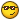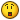# Any physics type dudes out there?

## Recommended Posts

I'm trying to use an AutoIt script to determine the estimated Rear Wheel Horsepower from a printout giving the variables of time, speed, distance, wheel circumference & mass. An example of the printout is:

Wheel = 1.825 m -- Mass = 285 kg

Time -- Speed -- Distance -- RPM -- Gear

0.248 -- 26.46 -- 1.8250 -- 3725 -- 1

0.529 -- 23.37 -- 3.6500 -- 3290 -- 1

0.760 -- 28.48 -- 5.4750 -- 4009 -- 1

0.961 -- 32.68 -- 7.3000 -- 4601 -- 1

1.138 -- 37.20 -- 9.1250 -- 5237 -- 1

1.299 -- 40.66 -- 10.950 -- 5724 -- 1

1.448 -- 44.03 -- 12.775 -- 6199 -- 1

1.588 -- 47.19 -- 14.600 -- 6644 -- 1

1.719 -- 50.09 -- 16.425 -- 7052 -- 1

1.843 -- 53.08 -- 18.250 -- 7473 -- 1

1.963 -- 54.67 -- 20.075 -- 7697 -- 1

2.076 -- 57.89 -- 21.900 -- 8150 -- 1

Speed is in kph and distance is in metres. I've checked out a few formulas I've found on the internet, but none of them seem to work (or I'm not using them correctly - take your pick). Maths was never my strong suite, so any help would be appreciated.

Thanks

P.S. Sorry, can't seem to get tabs to space the list correctlyEdit: used Gene's <spc><spc>--<spc><spc> tip on the list so it displays better.

Edited by PartyPooper
##### Share on other sites

I'm trying to use an AutoIt script to determine the estimated Rear Wheel Horsepower from a printout giving the variables of time, speed, distance, wheel circumference & mass. An example of the printout is:

Wheel = 1.825 m Mass = 285 kg

Time Speed Distance RPM Gear

0.248 26.46 1.825 3725 1

0.529 23.37 3.65 3290 1

0.76 28.48 5.475 4009 1

0.961 32.68 7.3 4601 1

1.138 37.2 9.125 5237 1

1.299 40.66 10.95 5724 1

1.448 44.03 12.775 6199 1

1.588 47.19 14.6 6644 1

1.719 50.09 16.425 7052 1

1.843 53.08 18.25 7473 1

1.963 54.67 20.075 7697 1

2.076 -- 57.89 -- 21.9 -- 8150 -- 1

Speed is in kph and distance is in metres. I've checked out a few formulas I've found on the internet, but none of them seem to work (or I'm not using them correctly - take your pick). Maths was never my strong suite, so any help would be appreciated.

Thanks

P.S. Sorry, can't seem to get tabs to space the list correctlyI made A s in Physics in college. but that was a long time ago, I'll look in to this, but I won't add anything to the thread unless I'm very confident.

When Tabs are unreliable, try SpaceSpaceDashDashSpaceSpace like I did with the last row of your table.

[font="Verdana"]Thanks for the response.Gene[/font]Yes, I know the punctuation is not right...

##### Share on other sites

I'm trying to use an AutoIt script to determine the estimated Rear Wheel Horsepower from a printout giving the variables of time, speed, distance, wheel circumference & mass. An example of the printout is:

Wheel = 1.825 m Mass = 285 kg

Time Speed Distance RPM Gear

0.248 26.46 1.825 3725 1

0.529 23.37 3.65 3290 1

0.76 28.48 5.475 4009 1

0.961 32.68 7.3 4601 1

1.138 37.2 9.125 5237 1

1.299 40.66 10.95 5724 1

1.448 44.03 12.775 6199 1

1.588 47.19 14.6 6644 1

1.719 50.09 16.425 7052 1

1.843 53.08 18.25 7473 1

1.963 54.67 20.075 7697 1

2.076 57.89 21.9 8150 1

Speed is in kph and distance is in metres. I've checked out a few formulas I've found on the internet, but none of them seem to work (or I'm not using them correctly - take your pick). Maths was never my strong suite, so any help would be appreciated.

Thanks

P.S. Sorry, can't seem to get tabs to space the list correctlyOn thinking about this I am surprised you didn't give a mass for the vehicle/wt beng moved. I looked up the Work & Power formulas in Machinery's Handbook 20th Edition and came to some results in MS Excel. However it seemd impractical to be just considering the Mass of the wheel. I also poked about a bit on the Internet. The following link takes you to an online calculator. It's units are English, but those are simple conversions.

http://www.speedworldmotorplex.com/calc.htm

Here are the formulas they use (in javascript), you can compare the results the page gives you with what you get from your interpretation of the formulas.

```function hp1() {
m = document.autocalc.trap.value/234;
w = document.autocalc.weight.value;
r = Math.pow(m,3)*w;
f = r * 1.18;
document.autocalc.result1.value = Math.round(r);
document.autocalc.result1a.value = Math.round(f);
}

function hp2() {
m = document.autocalc.et.value/5.825;
w2 = document.autocalc.weight2.value;
r2 = w2/Math.pow(m,3);
f2 = r2 * 1.18;
document.autocalc.result2.value = Math.round(r2);
document.autocalc.result2a.value = Math.round(f2);
}

function td1() {
wi = document.tire_diam.width.value/25.4;
s = (document.tire_diam.series.value/100)*2;
wd = 100*(document.tire_diam.wheel.value*1 + (wi * s));
r = Math.round(wd);
x = r/100;
document.tire_diam.result.value = x;
}

function mph() {
g = document.mph_rpm.gear.value*1;
td = document.mph_rpm.td.value*1;
x = td/(g*336);
document.mph_rpm.r10.value = (x * 1000);
document.mph_rpm.r15.value = (x * 1500);
document.mph_rpm.r20.value = (x * 2000);
document.mph_rpm.r25.value = (x * 2500);
document.mph_rpm.r30.value = (x * 3000);
document.mph_rpm.r35.value = (x * 3500);
document.mph_rpm.r40.value = (x * 4000);
document.mph_rpm.r45.value = (x * 4500);
document.mph_rpm.r50.value = (x * 5000);
document.mph_rpm.r55.value = (x * 5500);
document.mph_rpm.r60.value = (x * 6000);
document.mph_rpm.r65.value = (x * 6500);
document.mph_rpm.r70.value = (x * 7000);
document.mph_rpm.r75.value = (x * 7500);
}

function gr() {
a = document.gear.td2.value*1;
b = document.gear.rpm.value*1;
c = document.gear.speed.value*1;
ans = 100*((a*b)/(c*336));
ansr = Math.round(ans);

}```

[font="Verdana"]Thanks for the response.Gene[/font]Yes, I know the punctuation is not right...

##### Share on other sites

I did, Mass = 285 kgI'll check it out. Thanks.

I noticed the 285 kg, I was expecting more like a 1000, you must have been thinking motorcycle instead of car or truck.[font="Verdana"]Thanks for the response.Gene[/font]Yes, I know the punctuation is not right...

##### Share on other sites

Yes, it's a motorcycle. I built a speed sensor for the front wheel and now I'm trying to display the results using AutoIt. Having fun and learning more about AutoIt as I'm doing it##### Share on other sites

Yes, it's a motorcycle. I built a speed sensor for the front wheel and now I'm trying to display the results using AutoIt. Having fun and learning more about AutoIt as I'm doing itThat also makes the numbers I was getting make more sense. Because I wasn't thinking motorcycle, they seemed more than a little unreal.[font="Verdana"]Thanks for the response.Gene[/font]Yes, I know the punctuation is not right...

##### Share on other sites

Power = Work/Time

Work = Force*Distance

Force = Mass*Acceleration

Acceleration = (Change in Velocity / Change in Time)

so...

Power = (Mass*Velocity*Velocity)/Time (in Watts)

and since 1HP = 746Watts...

HP = (Mass*Velocity*Velocity)/(746*Time)

It's early in the morning and I haven't had any coffee yet, but the above equation should be what your looking for. Not trying to give the forum a physics lesson here, I just wrote it all out incase I made a mistake hopefully someone will catch it.

You need to convert your speeds from kph to m/s to use this formula (divide speed in kph by 3.6 to get m/s).

So for your first row of data, the HP calculation would be:

HP = (285*7.35*7.35)/(746*0.248) = 83.22HP

Similarly, for the second row it would be:

HP = (285*6.49*6.49)/(746*0.529) = 30.42HP

This will give you the instantaneous horsepower at each time interval. The horsepower profile for the data you gave would be as follows:

83.22

30.42

31.45

32.75

35.82

37.51

39.46

41.33

43.00

45.04

44.85

47.58

If these numbers look right to you, the equation is probably correct.

I'm off to get me a cup a' joe...

-DRX

## Create an account

Register a new account

• ### Recently Browsing   0 members

×

• Wiki

• Back

• #### Beta

• Git
• FAQ
• Our Picks
×
• Create New...